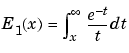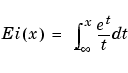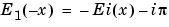MATLAB Function Referenceexpint

Exponential integral

Syntax

• ```Y = expint(X)
```

Definitions

The exponential integral computed by this function is defined as

•Another common definition of the exponential integral function is the Cauchy principal value integral

•which, for real positive `x`, is related to `expint` as

•Description

```Y = expint(X) ``` evaluates the exponential integral for each element of `X`.

References

  Abramowitz, M. and I. A. Stegun. Handbook of Mathematical Functions. Chapter 5, New York: Dover Publications, 1965.

© 1994-2005 The MathWorks, Inc.